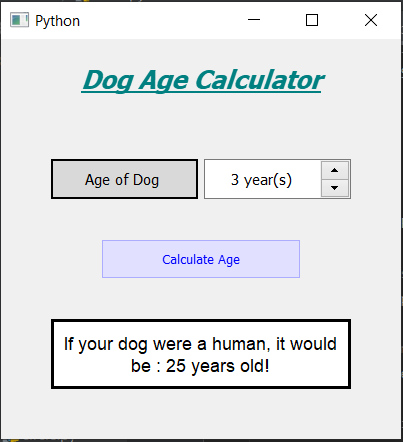# Dog Years Calculator using PyQt5

In this article, we will see how we can create a dog year calculator using PyQt5. Dog year calculator will tell the age of a dog if the dog was human, the dog grew a lot faster than humans. Below is how the calculator will look like :PyQt5 is a cross-platform GUI toolkit, a set of python bindings for Qt v5. One can develop an interactive desktop application with so much ease because of the tools and simplicity provided by this library. Below is the command to install the PyQt5

```pip install PyQt5
```

GUI Implementation Steps :

1. Create a heading label that displays the calculator name.
2. Create a label to show users to enter the age of the dog.
3. Create a QSpinBox object for the user to enter the years.
4. Create push-button for calculating the age.
5. Create a label to show the calculated age.

Back-End Implementation :

1. Add an action to the push button.
2. Set minimum and maximum values to the spin box.
3. Inside the push button, an action gets the value of spin box.
4. If the value is 1 then age is 15, if the value is 2 then age is 24 else age is increment by 4 for next years.
5. Show the calculated age with the help of a label.

Below is the implementation

## Python3

 `# importing required libraries ` `from` `PyQt5.QtWidgets ``import` `*`  `from` `PyQt5 ``import` `QtCore, QtGui ` `from` `PyQt5.QtGui ``import` `*`  `from` `PyQt5.QtCore ``import` `*`  `import` `datetime ` `import` `sys ` ` `  `# window class ` `class` `Window(QMainWindow): ` `   `  `    ``# Constructor  ` `    ``def` `__init__(``self``): ` `        ``super``().__init__() ` ` `  `        ``# setting title of the window ` `        ``self``.setWindowTitle(``"Python "``) ` ` `  `        ``# width of the window ` `        ``self``.w_width ``=` `400` ` `  `        ``# height of the window ` `        ``self``.w_height ``=` `400` ` `  `        ``# setting geometry of the window ` `        ``self``.setGeometry(``100``, ``100``, ``self``.w_width, ``self``.w_height) ` ` `  `        ``# method calling ` `        ``self``.UiComponents() ` ` `  `        ``# showing all the widgets ` `        ``self``.show() ` ` `  `    ``# method for components creation ` `    ``def` `UiComponents(``self``): ` `        ``# creating head label ` `        ``head ``=` `QLabel(``"Dog Age Calculator"``, ``self``) ` ` `  `        ``head.setWordWrap(``True``) ` ` `  `        ``# setting geometry of the head ` `        ``head.setGeometry(``0``, ``10``, ``400``, ``60``) ` ` `  `        ``# font work  ` `        ``font ``=` `QFont(``'Times'``, ``15``) ` `        ``font.setBold(``True``) ` `        ``font.setItalic(``True``) ` `        ``font.setUnderline(``True``) ` ` `  `        ``# setting font to the head ` `        ``head.setFont(font) ` ` `  `        ``# setting alignment of the head ` `        ``head.setAlignment(Qt.AlignCenter) ` ` `  `        ``# setting color effect to the head ` `        ``color ``=` `QGraphicsColorizeEffect(``self``) ` `        ``color.setColor(Qt.darkCyan) ` `        ``head.setGraphicsEffect(color) ` ` `  ` `  `        ``# creating a label ` `        ``age_label ``=` `QLabel(``"Age of Dog "``, ``self``) ` ` `  `        ``# setting geometry to the label ` `        ``age_label.setGeometry(``50``, ``120``, ``147``, ``40``) ` ` `  `        ``# setting alignment ` `        ``age_label.setAlignment(Qt.AlignCenter) ` ` `  `        ``# setting stylesheet ` `        ``age_label.setStyleSheet(``"QLabel"` `                                 ``"{"` `                                 ``"border : 2px solid black;"` `                                 ``"background : rgba(70, 70, 70, 35);"` `                                 ``"}"``) ` ` `  `        ``age_label.setFont(QFont(``'Times'``, ``9``)) ` ` `  `        ``# creating a spin box ` `        ``self``.age ``=` `QSpinBox(``self``) ` ` `  `        ``# setting geometry to the spin box ` `        ``self``.age.setGeometry(``203``, ``120``, ``147``, ``40``) ` ` `  `        ``# setting maximum value of spin box ` `        ``self``.age.setMaximum(``20``) ` ` `  `        ``# setting minimum value of spin box ` `        ``self``.age.setMinimum(``1``) ` ` `  `        ``# setting suffix to the spin box ` `        ``self``.age.setSuffix(``" year(s)"``) ` ` `  `        ``# setting font and alignment ` `        ``self``.age.setFont(QFont(``'Times'``, ``9``)) ` `        ``self``.age.setAlignment(Qt.AlignCenter) ` ` `  ` `  ` `  `        ``# creating a push button ` `        ``calculate ``=` `QPushButton(``"Calculate Age"``, ``self``) ` ` `  `        ``# setting geometry to the push button ` `        ``calculate.setGeometry(``100``, ``200``, ``200``, ``40``) ` ` `  `        ``# adding action to the button ` `        ``calculate.clicked.connect(``self``.calculate) ` ` `  `        ``# adding color effect to the push button ` `        ``color ``=` `QGraphicsColorizeEffect() ` `        ``color.setColor(Qt.blue) ` `        ``calculate.setGraphicsEffect(color) ` ` `  ` `  `        ``# creating a label to show result ` `        ``self``.result ``=` `QLabel(``self``) ` ` `  `        ``# setting properties to result label ` `        ``self``.result.setAlignment(Qt.AlignCenter) ` ` `  `        ``# setting geometry ` `        ``self``.result.setGeometry(``50``, ``280``, ``300``, ``70``) ` ` `  `        ``# making it multi line ` `        ``self``.result.setWordWrap(``True``) ` ` `  `        ``# setting stylesheet ` `        ``# adding border and background ` `        ``self``.result.setStyleSheet(``"QLabel"` `                                  ``"{"` `                                  ``"border : 3px solid black;"` `                                  ``"background : white;"` `                                  ``"}"``) ` ` `  `        ``# setting font ` `        ``self``.result.setFont(QFont(``'Arial'``, ``11``)) ` ` `  ` `  `    ``# method for calculating the dog's age ` `    ``def` `calculate(``self``): ` ` `  `        ``# getting the spin box value ` `        ``value ``=` `self``.age.value() ` ` `  `        ``# if value is 1 ` `        ``if` `value ``=``=` `1``: ` ` `  `            ``# dog age is 15 ` `            ``d_age ``=` `15` ` `  `        ``# if value is 2 ` `        ``elif` `value ``=``=` `2``: ` ` `  `            ``# dog age is 24 ` `            ``d_age ``=` `24` ` `  `        ``# else dog age get incremented by 4 ` `        ``else``: ` `            ``d_age ``=` `24` `+` `(value ``-` `2``) ``*` `4` ` `  ` `  `        ``# showing age through label ` `        ``self``.result.setText(``"If your dog were a human, it would be : "` `+``str``(d_age) ``+` `" years old !"``) ` ` `  ` `  ` `  `# create pyqt5 app ` `App ``=` `QApplication(sys.argv) ` ` `  `# create the instance of our Window ` `window ``=` `Window() ` ` `  `# start the app ` `sys.exit(App.``exec``())`

Output :

My Personal Notes arrow_drop_upCheck out this Author's contributed articles.

If you like GeeksforGeeks and would like to contribute, you can also write an article using contribute.geeksforgeeks.org or mail your article to contribute@geeksforgeeks.org. See your article appearing on the GeeksforGeeks main page and help other Geeks.

Please Improve this article if you find anything incorrect by clicking on the "Improve Article" button below.

Article Tags :

Be the First to upvote.

Please write to us at contribute@geeksforgeeks.org to report any issue with the above content.